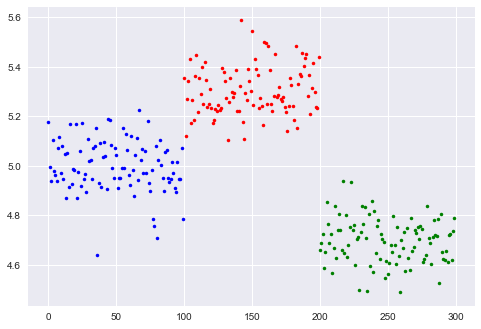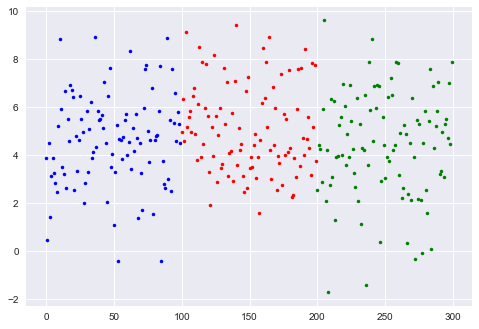# One-way ANOVA¶

For the one-way ANOVA, you can use either the function in "scipy.stats". Alternatively, you can use the "statsmodel" tools: they provide more, and nicer formatted, information.

Author: Thomas Haslwanter, Date: Feb-2017

In :
%pylab inline
import scipy.stats as stats
import pandas as pd
import urllib
from statsmodels.formula.api import ols
from statsmodels.stats.anova import anova_lm
import seaborn as sns

Populating the interactive namespace from numpy and matplotlib


# The importance of the variance¶

In :
centers = [5, 5.3, 4.7]
std1 = 0.1
colors = 'brg'

data1 = []
for ii in range(3):
data1.append(stats.norm(centers[ii], std1).rvs(100))
plot(arange(len(data1[ii]))+ii*len(data1), data1[ii], '.', color=colors[ii])In :
std2 = 2
data2 = []
for ii in range(3):
data2.append(stats.norm(centers[ii], std2).rvs(100))
plot(arange(len(data1[ii]))+ii*len(data2), data2[ii], '.', color=colors[ii])Note: In both cases the means have the same difference, but the variance is much larger in data2!

## ANOVA with Sample Data¶

### Get and sort sample data¶

Twenty-two patients undergoing cardiac bypass surgery were randomized to one of three ventilation groups:

• Group I: Patients received 50% nitrous oxide and 50% oxygen mixture continuously for 24 h.
• Group II: Patients received a 50% nitrous oxide and 50% oxygen mixture only dirng the operation.
• Group III: Patients received no nitrous oxide but received 35-50% oxygen for 24 h.

The data show red cell folate levels for the three groups after 24h' ventilation.

In :
# Get the data
inFile = 'altman_910.txt'
url_base = 'https://raw.githubusercontent.com/thomas-haslwanter/statsintro_python/master/ipynb/Data/data_altman/'

url = url_base + inFile
data = genfromtxt(urllib.request.urlopen(url), delimiter=',')

# Sort them into groups, according to column 1
group1 = data[data[:,1]==1,0]
group2 = data[data[:,1]==2,0]
group3 = data[data[:,1]==3,0]


### Levene-test¶

A Levene-test and/or a normality test should be made before applying a oneway ANOVA.

In :
# check if the variances are equal with the "Levene"-test
(W,p) = stats.levene(group1, group2, group3)
if p<0.05:
print('Warning: the p-value of the Levene test is <0.05: p={0}'.format(p))

Warning: the p-value of the Levene test is <0.05: p=0.045846812634186246


### One-way ANOVA¶

In :
F_statistic, pVal = stats.f_oneway(group1, group2, group3)

print('The results from the one-way ANOVA, with the data from Altman 910: F={0:.1f}, p={1:.5f}'.format(F_statistic, pVal))
if pVal < 0.05:
print('One of the groups is significantly different.')

The results from the one-way ANOVA, with the data from Altman 910: F=3.7, p=0.04359
One of the groups is significantly different.


## Elegant alternative implementation, with pandas & statsmodels¶

In :
df = pd.DataFrame(data, columns=['value', 'treatment'])

# the "C" indicates categorical data
model = ols('value ~ C(treatment)', df).fit()

print(anova_lm(model))

                df        sum_sq      mean_sq         F    PR(>F)
C(treatment)   2.0  15515.766414  7757.883207  3.711336  0.043589
Residual      19.0  39716.097222  2090.320906       NaN       NaN

C:\Programs\WinPython-64bit-3.6.0.1Qt5\python-3.6.0.amd64\lib\site-packages\scipy\stats\_distn_infrastructure.py:875: RuntimeWarning: invalid value encountered in greater
return (self.a < x) & (x < self.b)
C:\Programs\WinPython-64bit-3.6.0.1Qt5\python-3.6.0.amd64\lib\site-packages\scipy\stats\_distn_infrastructure.py:875: RuntimeWarning: invalid value encountered in less
return (self.a < x) & (x < self.b)
C:\Programs\WinPython-64bit-3.6.0.1Qt5\python-3.6.0.amd64\lib\site-packages\scipy\stats\_distn_infrastructure.py:1814: RuntimeWarning: invalid value encountered in less_equal
cond2 = cond0 & (x <= self.a)